# Generating Random Integers With Arbitrary Probabilities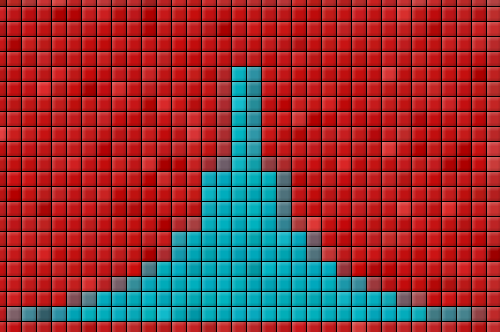I finally laid my hands on Donald Knuth’s The Art of Computer Programming (what a wonderful set of books!), and found a neat algorithm for generating random integers 0, 1, 2, … , n – 1, with probabilities p_0, p_1, … , p_(n-1).

I have written about generating random numbers (floats) with arbitrary distributions for one dimension and higher dimensions, and indeed that method can be adapted for generating integers with specific probabilities. However, the method described below is much more concise, and efficient (I would guess) for this special case. Moreover, it is also easy to adapt it to generate floats for continuous distributions.

## Description of the Algorithm

The basic idea of the algorithm is simple. We have two tables of length n that contains integers (K, L), and a third table that contains probabilities (P). The first table merely contains the integers 0 to n-1 (thus, we need not actually store it explicitly). The other two tables are computed before generation (more about that below).

To generate a random number, we generate a random integer (uniformly distributed between 0 and n-1 inclusive), and a random float (uniformly distributed between 0 and 1). The integer tells us which cells in the table to use. First we lookup the probability in P at that index. If the float is smaller than that value, we return the integer in K, otherwise we return the integer in L. (Note, the integer in K is exactly the random integer itself. Therefore, we do not actually have a table K – we simply use the random integer.)

Now this will only give the desired result if the tables have been constructed for this to work. Before looking at how these tables are generated, let us look at a very simple example. Suppose we want to generate 0, 1, 2, with probabilities 3/18, 7/18, 8/18. Can you see why the following tables will work?

 0 1 2 P 1/2 1 5/6 L 2 * 1

There is only one way to generate 0: if the random integer is 0, and the random float is below 1/2. This will happen with probability 1/3 x 1/2 = 1/6 = 3/18.

There are two ways of generating 1:

• if the random integer is 1 (probability 1/3 = 6/18); or
• if the random integer is 2, and the float is above 5/6 (probability 1/3 x 1/6 = 1/18).

Adding these probabilities, we get 6/18 + 1/18 = 7/18.

There are also two ways to generate 2:

• if the random integer is 0 and the random float is above 1/2 (1/3 x 1/2 = 1/6 = 3/18); or
• if the random integer is 2 and the random float is below 5/6 (1/3 x 5/6 = 5/18).

Adding these probabilities, we get 3/18 + 5/18 = 8/18.

## Generating the tables

Consider this problem:

• We have n squares that we want to paint.
• We have five colours of paint, possibly different amounts of paint for each colour.
• Each square has a border in one of the colours; no two borders are the same colour.
• In total, there is just enough paint to cover the n squares exactly.
• We want to paint each square with at most two colours, with one colour matching the border.

To do this, we sort the paint buckets in ascending order. We paint the square that matches the first bucket with the first bucket, and whatever remains with the last bucket. The first bucket is empty (why?), and there might be some paint remaining in the last bucket. We now put this bucket back so that the buckets are sorted according to the new quantities of paint. The first square is completely covered (why?). Note that the painted square’s colour corresponds with the depleted colour.

The situation is now: we have n-1 unpainted squares, and n-1 colours of paint. This is the same problem as the initial problem, with one less square and one less colour. Therefore, we proceed as before, and repeat this process until all the squares have been painted and all the paint has been used.

To answer the two why’s above:

The first bucket is always empty, because the smallest bucket cannot cover more than one square. Since it is the smallest bucket, all other buckets must contain at least as much paint. Thus, we have n colours, and enough paint of each colour to paint more than one square. Thus, in total, we must have more paint than is required to paint n squares. But we said that we have exactly the right amount of paint needed, not more. Therefore, the smallest amount of paint cannot cover more than one square.

The first square is always covered completely, since the last bucket always contains enough paint for at least one square. If it did not, since it is the largest bucket, all other buckets will paint less than one square. In total, we would have n colours, each that can cover less than one square. Thus, all our paint will cover less than n squares: we do not have enough paint. But we said that we do, so this cannot be. Therefore, we must have enough paint in the last bucket to cover at least one square.

Below is an illustration of this process for three colours. Here we assume that 1 litre of paint covers 1 square. We have 1/3 = 3/6 litre of red, 7/6 litre of green, and 8/6 litre of cyan.

### Sort Paint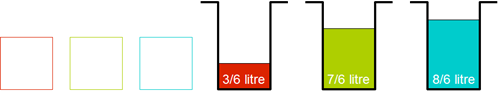### Paint from first bucket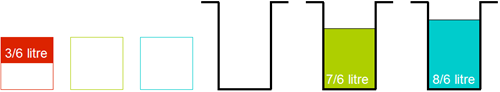### Paint from last bucket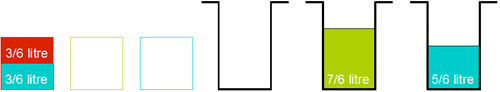### Sort Paint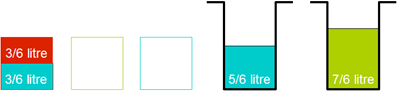### Paint from first bucket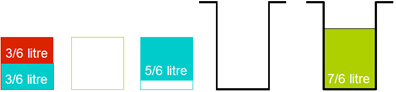### Paint from last bucket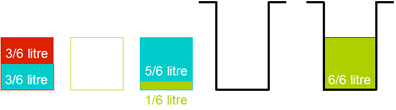### Sort paint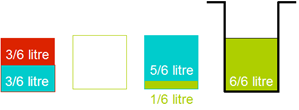### Paint from first bucket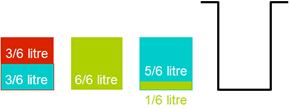As you can see, the solution above corresponds with the tables given in the example above. Indeed, the algorithm for calculating the tables is exactly the same as the paint algorithm:

• The n colours correspond to the n integers (from 0 to n-1) that we want to generate.
• The initial amounts of paints corresponds with the (relative) probabilities that we want to generate each integer.
• The amount of paint used to paint a square of the same border is the entry in table P – the probability of using the number associated with that cell (i.e., “the border”).
• The other colour used to paint a square (if any) corresponds to the entry in table K.

## A Small Optimisation in the Implementation

### Generating two uniform random numbers for the price of one

There is a trick to generate a random integer (0 <= n < k) and a random float (0 <= x < 1) from a single random float (0 <= u < 1) that is used in the implementation of this algorithm (see download below).

The trick is:

• n = floor (uk)
• x = uk – n

This assumes things about the random generator and the accuracy required. (I do not want to get into the details here).